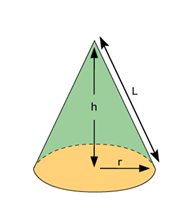# Volume of a Cone Formula

Cone is a 3D structure having a circular base and an apex/vertex. Every point on the circular base is connected to the apex by a set of line segments. A cone can be viewed as a stack of non-congruent circular discs that are placed one above the other such that the ratio of the diameter of the adjacent discs remains constant. The volume of a cone is its capacity or is the amount of space occupied by the cone. It can be mathematically expressed as:

$$Volume\ of\ Cone =\frac{1}{3}\pi r^{2}h$$
Where r is the base radius and h is the height of the cone.## Derivation:

Here’s an activity to compute the volume of a cone.

• Take a conical flask and a cylindrical container of same base radius and same height.
• Keep adding water to the flask until it is filled to the brim. Start pouring this water to the cylindrical container. You will observe that it does not fill up the cylinder completely.
• Again fill the conical flask with water to the brim. Now add this water to the cylinder, you will observe that there is still some vacant space in the container.
• Perform this experiment yet once again. This time you will notice that the cylindrical container is entirely filled.

We can conclude from this experiment that the volume of a cylinder is thrice the volume of a cone having the same height and base radius. This implies that the volume of a cone is equal to one-third of the volume of the cylinder having the same height and base radius.

Now, you know that volume of a cylinder = $$\pi r^{2}h$$

Hence,

Volume of a cone = $$\frac{1}{3}\pi r^{2}h$$

Volume of Cone in Real Life:

In our everyday life, we come across different types of cones. The volume of cone formula can be used in manufacturing and calculating the capacity or volume of traffic cones, ice-cream cones, funnels and even the regular cylinders.  You can easily find out the volume of a cone if you have the measurements of its height and radius.

### Solved Examples

Question 1: Find the volume of a cone, if the radius is 4 cm and height is 9 cm.

Solution:

Height h = 9 cm

Using the volume of a cone formula,

Volume of cone = $$\frac{1}{3}\pi r^{2}h$$

Volume of cone = 1/3 x 3.14 x 4² x 9 = 150.72 cm³

Question 2: Find the volume of a cone which has a base radius of 8 cm and slant height (l) of 13 cm.

Solution: Given,

Slant height l = 13 cm

Slant height, $$l=\sqrt{h^{2}+r^{2}}$$

$$13^{2}=h^{2}+8^{2}$$

$$h^{2}=13^{2}-8^{2}$$

$$h=\sqrt{13^{2}-8^{2}}=\sqrt{169-64}=10.24 cm$$

Using the formula of volume of cone,

Volume of cone = $$\frac{1}{3}\pi r^{2}h$$

Volume of cone = $$\frac{1}{3}\times 3.14\times 8^{2}\times 10.24=685.94cm^{3}$$

To solve more problems on the topic, download BYJU’S – The Learning App.